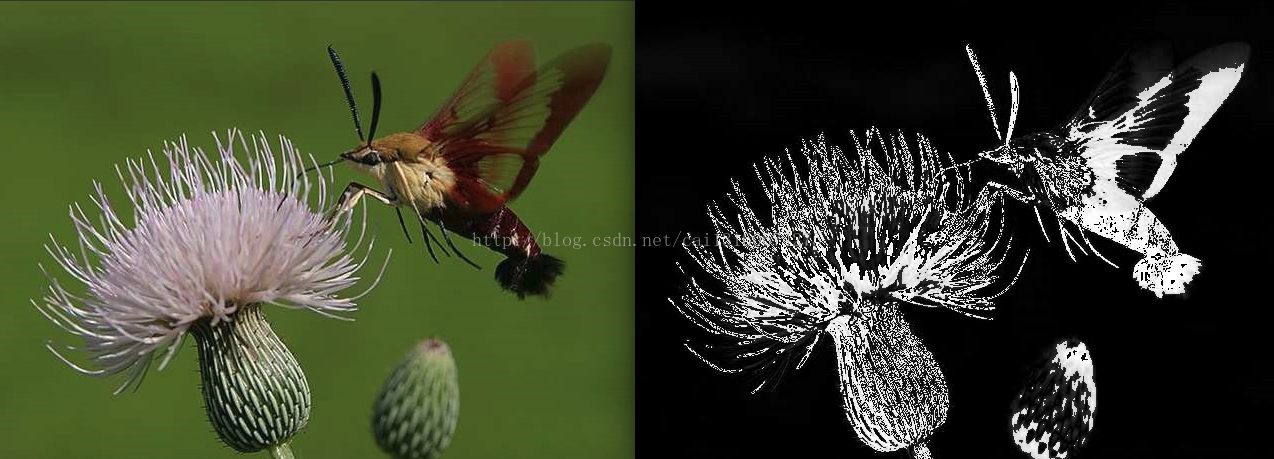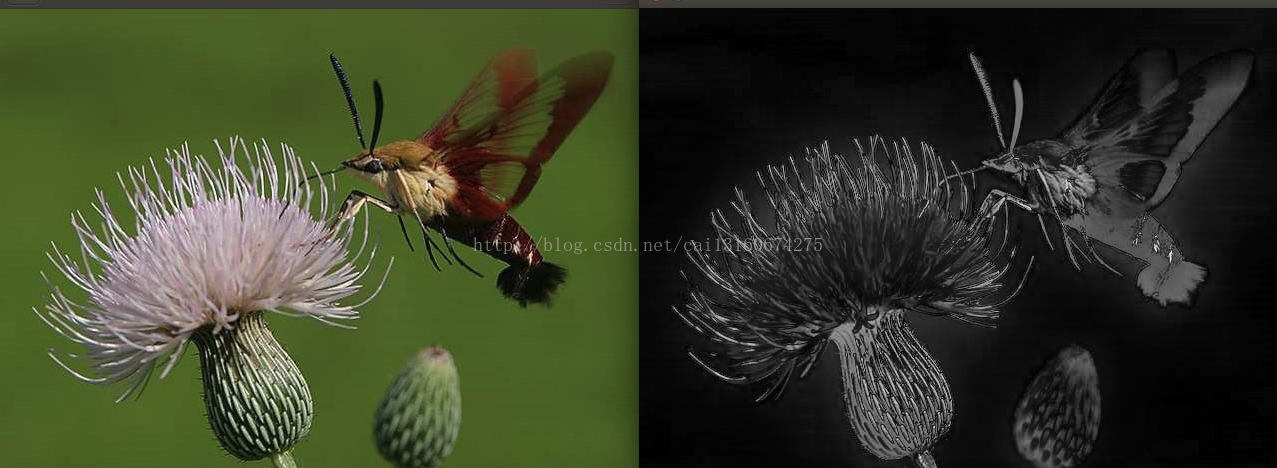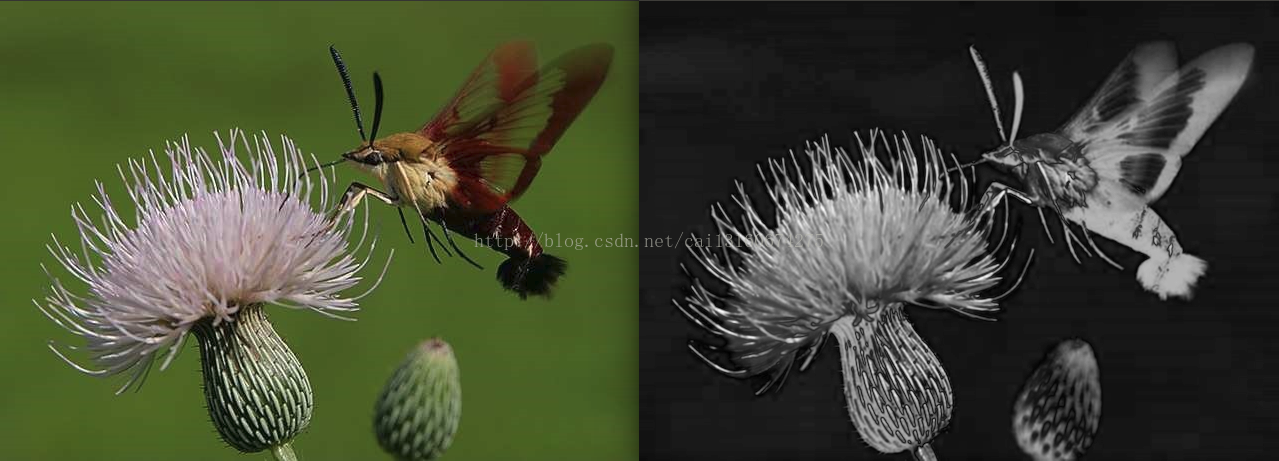• 显著性检测(saliency detection)评价指标之NSS的Matlab代码实现
2021-04-21 03:28:50

calcNSSscore.m

function [ score ] = calcNSSscore( salMap, eyeMap )

%calcNSSscore Calculate NSS score of a salmap

% Usage: [score] = calcNSSscore ( salmap, eyemap )

%

% score : an array of score of each eye fixation

% salmap : saliency map. will be resized nearest neighbour to eyemap

% eyemap : should be a binary map of eye fixation

% clear;clc;

% eyeMap=rgb2gray(eyeMap);

%%% Resize and normalize saliency map

salMap = double(imresize(salMap,size(eyeMap),'bicubic'));

mapMean = mean2(salMap); mapStd = std2(salMap);

salMap = (salMap - mapMean) / mapStd; % Normalized map

%%% NSS calculation

[X Y] = find(eyeMap > 0);

NSSVector = zeros(1,size(X,1));

for p=1:size(X,1)

NSSVector(p) = salMap(X(p),Y(p));

end

score = NSSVector;

% end

main.m

clear;

clc;

smap_path='E:\Dataset180303\final_data\smap_Result1\';

gmap_path='E:\Dataset180303\final_data\image_resize_gt\';

smap_file=dir(smap_path);

% for i=3:length(vedio_file)

% disp(i);

% vedio_name=strcat(smap_path,vedio_file(i).name);%字符串

% smap_file=dir(vedio_name);%文件夹

%

% vedio_name1=strcat(gmap_path,vedio_file(i).name);

% gmap_file=dir(vedio_name1);

for j=3:length(smap_file)

disp(j-2);

gmap_name=strcat(gmap_path,num2str(j-2), '.jpg');

% gmap_name=strcat(gmap_path,smap_file(j).name);

% smap_name=strcat(smap_path,num2str(j-2+ 0 ), '_SaliencyMap', '.jpg');

smap_name=strcat(smap_path,num2str(j-2 +0), '.jpg');

sal_map=mat2gray(smap);

if size(gmap,3)==3

gt_map=rgb2gray(gmap);

else

gt_map=gmap;

end

threshold_value = graythresh(gt_map);%使用最大类间方差法：找到图片的一个合适的阈值(threshold)。

% threshold_value=0.01;

gt_final_map = im2bw(gt_map, threshold_value);%make gt_map to boolean map也叫逻辑矩阵

b=calcNSSscore(sal_map,gt_final_map);

b = abs(b);

a(j-2,1)=mean(b);

disp(j-2);

end

idx=find(isnan(a));

a(idx)=1.5;

RGBD_NSS=mean(a);

% clear a;

% end

nss = mean(RGBD_NSS);

更多相关内容
• 简单图像显著特征提取matlab代码，代码仅有几行，很容易实现,效果比较明显。
• fps=1上文讲了几种简单的方法，显著性检测就是把一幅图像中最吸引人注意的部分提取出来。我用opencv重写了LC，AC,FT三种算法，代码和效果如下：1.，后面的方法其实大概都是基于这个实现的，代码样子差不多LC思路就是...

https://blog.csdn.net/cai13160674275/article/details/72991049?locationNum=7&fps=1

上文讲了几种简单的方法，显著性检测就是把一幅图像中最吸引人注意的部分提取出来。

我用opencv重写了LC，AC,FT三种算法，代码和效果如下：

1.，后面的方法其实大概都是基于这个实现的，代码样子差不多

LC思路就是利用对某个像素点累加其与全幅像素的距离(欧式距离)，然后归一化到0-255，由于是rgb信息，于是用直方图优化，提前计算好每个颜色与其他的距离和

void SalientRegionDetectionBasedonLC(Mat &src){

int HistGram={0};

int row=src.rows,col=src.cols;

int gray[row][col];

//int Sal_org[row][col];

int val;

Mat Sal=Mat::zeros(src.size(),CV_8UC1 );

Point3_* p;

for (int i=0;i

for (int j=0;j

p=src.ptr > (i,j);

val=(p->x + (p->y) *2 + p->z)/4;

HistGram[val]++;

gray[i][j]=val;

}

}

int Dist;

int Y,X;

int max_gray=0;

int min_gray=1<<28;

for (Y = 0; Y

{

val = 0;

for (X = 0; X

val += abs(Y - X) * HistGram[X];                //    论文公式(9)，灰度的距离只有绝对值，这里其实可以优化速度，但计算量不大，没必要了

Dist[Y] = val;

max_gray=max(max_gray,val);

min_gray=min(min_gray,val);

}

for (Y = 0; Y

{

for (X = 0; X

{

Sal.at(Y,X) = (Dist[gray[Y][X]] - min_gray)*255/(max_gray - min_gray);        //    计算全图每个像素的显著性

//Sal.at(Y,X) = (Dist[gray[Y][X]])*255/(max_gray);        //    计算全图每个像素的显著性

}

}

imshow("sal",Sal);

waitKey(0);

}

效果图2.AC算法也挺有意思，利用了类似图像金字塔算法，在不同纬度(具体实现是用大小不同的均值滤波器过滤图像)与标准图像做差并累加，然后归一化，实现如下:

void SalientRegionDetectionBasedonAC(Mat &src,int MinR2, int MaxR2,int Scale){

Mat Lab;

cvtColor(src, Lab, CV_BGR2Lab);

int row=src.rows,col=src.cols;

int Sal_org[row][col];

memset(Sal_org,0,sizeof(Sal_org));

Mat Sal=Mat::zeros(src.size(),CV_8UC1 );

Point3_* p;

Point3_* p1;

int val;

Mat filter;

int max_v=0;

int min_v=1<<28;

for (int k=0;k

int len=(MaxR2 - MinR2) * k / (Scale - 1) + MinR2;

blur(Lab, filter, Size(len,len ));

for (int i=0;i

for (int j=0;j

p=Lab.ptr > (i,j);

p1=filter.ptr > (i,j);

//cout<x - p1->x)*(p->x - p1->x)+ (p->y - p1->y)*(p->y-p1->y) + (p->z - p1->z)*(p->z - p1->z) <

val=sqrt( (p->x - p1->x)*(p->x - p1->x)+ (p->y - p1->y)*(p->y-p1->y) + (p->z - p1->z)*(p->z - p1->z) );

Sal_org[i][j]+=val;

if(k==Scale-1){

max_v=max(max_v,Sal_org[i][j]);

min_v=min(min_v,Sal_org[i][j]);

}

}

}

}

cout<

int X,Y;

for (Y = 0; Y

{

for (X = 0; X

{

Sal.at(Y,X) = (Sal_org[Y][X] - min_v)*255/(max_v - min_v);        //    计算全图每个像素的显著性

//Sal.at(Y,X) = (Dist[gray[Y][X]])*255/(max_gray);        //    计算全图每个像素的显著性

}

}

imshow("sal",Sal);

waitKey(0);

}

SalientRegionDetectionBasedonAC(test,test.rows/8,test.rows/2,3);3.FT算法

lab空间的均值减去当前像素值

void SalientRegionDetectionBasedonFT(Mat &src){

Mat Lab;

cvtColor(src, Lab, CV_BGR2Lab);

int row=src.rows,col=src.cols;

int Sal_org[row][col];

memset(Sal_org,0,sizeof(Sal_org));

Point3_* p;

int MeanL=0,Meana=0,Meanb=0;

for (int i=0;i

for (int j=0;j

p=Lab.ptr > (i,j);

MeanL+=p->x;

Meana+=p->y;

Meanb+=p->z;

}

}

MeanL/=(row*col);

Meana/=(row*col);

Meanb/=(row*col);

GaussianBlur(Lab,Lab,Size(3,3),0,0);

Mat Sal=Mat::zeros(src.size(),CV_8UC1 );

int val;

int max_v=0;

int min_v=1<<28;

for (int i=0;i

for (int j=0;j

p=Lab.ptr > (i,j);

val=sqrt( (MeanL - p->x)*(MeanL - p->x)+ (p->y - Meana)*(p->y-Meana) + (p->z - Meanb)*(p->z - Meanb) );

Sal_org[i][j]=val;

max_v=max(max_v,val);

min_v=min(min_v,val);

}

}

cout<

int X,Y;

for (Y = 0; Y

{

for (X = 0; X

{

Sal.at(Y,X) = (Sal_org[Y][X] - min_v)*255/(max_v - min_v);        //    计算全图每个像素的显著性

//Sal.at(Y,X) = (Dist[gray[Y][X]])*255/(max_gray);        //    计算全图每个像素的显著性

}

}

imshow("sal",Sal);

waitKey(0);

}四种比较简单的图像显著性区域特征提取方法原理及实现-----&gt&semi; AC&sol;HC&sol;LC&sol;FT。

laviewpbt  2014.8.4 编辑 Email:laviewpbt@sina.com   QQ:33184777 最近闲来蛋痛,看了一些显著性检测的文章,只是简单的看看,并没有深入的研究,以 ...

四种简单的图像显著性区域特征提取方法-----AC&sol;HC&sol;LC&sol;FT。

四种简单的图像显著性区域特征提取方法-----> AC/HC/LC/FT. 分类: 图像处理 2014-08-03 12:40 4088人阅读 评论(4) 收藏 举报 salient regio ...

图像局部显著性—点特征(SURF)

1999年的SIFT(ICCV 1999,并改进发表于IJCV 2004,本文描述):参考描述:图像特征点描述. 参考原文:SURF特征提取分析 本文有大量删除,如有疑义,请参考原文. SURF对SI ...

基于qml创建最简单的图像处理程序(3)-使用opencv&amp&semi;qml进行图像处理

系列课程及配套代码基于qml创建最简单的图像处理程序(1)-基于qml创建界面http://www.cnblogs.com/jsxyhelu/p/83 ...

Python下opencv使用笔记(二)(简单几何图像绘制)

简单几何图像一般包含点.直线.矩阵.圆.椭圆.多边形等等.首先认识一下opencv对像素点的定义. 图像的一个像素点有1或者3个值.对灰度图像有一个灰度值,对彩色图像有3个值组成一个像素值.他们表现出 ...

图像局部显著性—点特征(GLOH)

基于古老的Marr视觉理论,视觉识别和场景重建的基础即第一阶段为局部显著性探测.探测到的主要特征为直觉上可刺激底层视觉的局部显著性--特征点.特征线.特征块. 相关介绍:局部特征显著性-点特征(SIF ...

图像显著性论文(一)—A Model of saliency Based Visual Attention for Rapid Scene Analysis

这篇文章是图像显著性领域最具代表性的文章,是在1998年Itti等人提出来的,到目前为止引用的次数超过了5000,是多么可怕的数字,在它的基础上发展起来的有关图像显著性论文更是数不胜数,论文的提出主要 ...

Python用Pillow&lpar;PIL&rpar;进行简单的图像操作

Python用Pillow(PIL)进行简单的图像操作 颜色与RGBA值 计算机通常将图像表示为RGB值,或者再加上alpha值(通透度,透明度),称为RGBA值.在Pillow中,RGBA的值表示为 ...

图像的全局特征--HOG特征、DPM特征

HOG特征:方向梯度直方图(Histogram of Oriented Gradient,)特征是一种全局图像特征描述子. 它通过计算和统计图像局部区域的梯度方向直方图来构成特征.Hog特征结合SVM ...

随机推荐

Java jstatd详解

jstatd 命令全称: Virtual Machine jstat Daemon DESCRIPTION The  jstatd tool is an RMI server application ...

视觉中的深度学习方法CVPR 2012 Tutorial Deep Learning Methods for Vision

Deep Learning Methods for Vision CVPR 2012 Tutorial  9:00am-5:30pm, Sunday June 17th, Ballroom D (Fu ...

RPi 2B Documentation

/********************************************************************** * RPi 2B Documentation * 声明: ...

帝国cms7&period;0 内容页控制简介字数！

帝国cms7.0 内容页有简介部分,使用以下代码可以有效控制字数限制! 下载类简介:=esub($navinfor[softsay],160)?> 文章类简介:=esub($ ...

python TCP编程

1.socket 服务端和客户端通过socket套接字进行通信 2.服务端 import socket import threading def tcp_handler(connect_sock, a ...

PCL中分割方法的介绍(3)

(3)上两篇介绍了关于欧几里德分割,条件分割,最小分割法等等还有之前就有用RANSAC法的分割方法,这一篇是关于区域生成的分割法, 区 域生长的基本 思想是: 将具有相似性的像素集合起来构成区域.首先 ...

顾名思义,装载器就是加载元素的,使用CI时,经常加载的有: 加载类库文件:$this->load->library() 加载视图文件:$this->load->view() ...

Windows 2003扩充磁盘空间

diskpartlist volumeselect volume 1 (选择需要扩充空间的分区)extend size=409600 (单位为MB)

python笔记2 生成器 文件读写

生成器 一边循环一边计算的机制,称为生成器(Generator). 把一个列表生成式的[]改成(),就创建了一个generator: 创建了一个generator后,通过for循环来迭代它. 著名的斐 ...

Balanced Numbers(数位dp)

Description Balanced numbers have been used by mathematicians for centuries. A positive integer is c ...

展开全文• 显著性检测AC的matlab实现，测试可用。包括AC.m ， RGB2lab.m，论文。
• Itti模型作为图像显著区域识别系统的模型；matlab语言实现；最后得出亮度显著图 、颜色显著图 及方向显著
• FT显著性算法matlab代码，代码简单明了，亲测效果很好
• 图像的显著区域能代表图像的主要内容，提取图像的显著区域在图像检索、敏感图像识别等方面有重要的作用。结合多尺度分析先提取图像的显著图，而后在显著图的基础上根据经验阈值对事先的分割区域进行判断取舍，最后...
• 分别对显著区域和非显著区域进行特征提取，具体提取特征包括颜色(Lab空间，即三个分量)，纹理，方向等，最后每幅图片用两个特征向量表示（一个显著区域特征向量，一个非显著区域特征向量）。 根据上面第一部分...

分别对显著区域和非显著区域进行特征提取，具体提取的特征包括颜色(Lab空间，即三个分量)，纹理，方向等，最后每幅图片用两个特征向量表示（一个显著区域特征向量，一个非显著区域特征向量）。

根据上面第一部分得到的结果，每个图像对应这一个显著性区分图，即：

根据步骤一的结果，我们进行特征提取：这里，根据要求，主要的特征为颜色(Lab空间，即三个分量)，纹理，方向等，为了提高精度，我们增加其余几种特征，主要为：

这里，我们考虑其四个方向上的四个文理参数的均值和方差作为最终的特征参数，此外，再加上LAB空间的颜色分量，总共这些均作为其特征参数。

颜色特征，

这里根据要求，使用lab特征空间，最后使用的是计算均值作为三个特征数据。关于这个部分的内容，你可以找一些lab的理论用在论文中。

关于纹理特征：

(1)能量：即上述特征量中的角二阶矩，是灰度共生矩阵中各个元素的平方和，该特征量可以描述图像的灰度分布情况以及纹理粗细度，是纹理灰度变化的度量特征量。如果图像纹理粗糙，则该特征量较大，反之，纹理细腻，则该特征量较小。

(2)对比度：是灰度共生矩阵中主对角线的惯性矩，该特征量是图像清晰程度以及纹理沟纹深浅程度的度量特征量。对比度值较小，说明图像效果清晰，沟纹较浅，反之，对比度值较大，说明图像效果模糊，沟纹较深。

(3)相关：是灰度共生矩阵行或者列方向上元素相似程度的度量特征量，该特征量可以作为判断纹理主方向的特征量。

(4)熵：图像信息量，是一个随机性的度量特征量，当灰度共生矩阵中元素分散程度较大时候，熵较大，图像为满纹理时候，熵取得最大值，反之如果纹理分布较集中，则熵较小，如果图像中无纹理，则熵取最小值零。

我们选择上述常用的四个纹理特征的均值和方差作为特征值。具体公式，请查看相关论文即可。

我们分别从0,45,90,135四个方向计算其纹理空间。

3、赋予显著区域特征向量高权值，非显著区域特征向量低权值。（具体做法请你们自己解决，我有个思路，你们可以参考一下不知道可不可行，就是以显著区域和非显著区域像素点个数的倒数，进行归一化，然后作为权值，因为一般情况下显著区域肯定比非显著区域面积要小，即像素个数少，求倒数之后，则显著区域的权值自然比非显著区域的大），这部分务必写清楚具体实现步骤，论文里需要写。

4、然后用支持向量机的方法对Corel5k图像库的图片进行训练，得出模型，用于对未知图片进行标注。(具体写清楚用的那个类型支持向量机，对应的原始公式等等)

支持向量机(Support Vector Machine)是Cortes和Vapnik于1995年首先提出的，它在解决小样本、非线性及高维模式识别中表现出许多特有的优势，并能够推广应用到函数拟合等其他机器学习问题中。支持向量机方法是建立在统计学习理论的VC 维理论和结构风险最小原理基础上的，根据有限的样本信息在模型的复杂性（即对特定训练样本的学习精度，Accuracy）和学习能力（即无错误地识别任意样本的能力）之间寻求最佳折衷，以期获得最好的推广能力（或称泛化能力）。

展开全文• 资源是ITTI视觉显著性模型的MATALB代码，资源下载后直接能用，解压后打开zj.m，然后运行即可测试，提取得到的显著图直接能显示出来。 同时为了大家需要，同时考虑了灰度图像可以使用的边缘显著度等，去掉了与颜色...
• 资源是SR显著提取算法的MATLAB代码，资源下载后直接能用，解压后打开test.m，然后运行即可。相关的资料请参考博文https://blog.csdn.net/weixin_42647783/article/details/81415480，谢谢~
• 显着图提取的代码matlab 用于 3D fMRI 体积分类的 3D 卷积神经网络 (CNN) 这些是用于 3D fMRI 体积分类的 3D-CNN 模型的 MATLAB 代码和 Python 代码。 还包括可视化经过训练的 3D-CNN 的代码。 样本数据： 样本 3D ...
• 这种存储方式，在matlab就叫做full storage organization。 % 2.只存储非零元素，那么怎么存储呢？ % (4,2) 1 % (2,3) 1 % (4,4) 2 % 最优化关联矩阵 (公式3) f∗ = y/(D − αW) . dd = sum(W); %sum函数不写第二个...

本代码内容是关于Saliency Detection via Graph-Based Manifold Ranking的算法详解，想要运行此代码还需要一系列的文件，单纯此代码无法演示结果的。

可以在网上搜索完整文件，简单来说就是基本的SLIC小程序，其余的不是必要项。将数据集直接放入test文件夹中，运行sample或者demo都可以吧，我的是师兄传给我的，跟各位下载的结果应该差别不大。

(为了避免大家浪费时间，这里贴出百度云下载链接，链接：

关注并且私信我发链接

clear all;close all;clc;

%%------------------------设置参数---------------------%%

theta = 0.1; % 控制边缘的权重

alpha = 0.99; % 控制流行排序成本函数两个项的平衡

spnumber = 200; % 超像素的数量

imgRoot = './test/'; % 测试图像的路径

saldir = './saliencymap/'; % 显著性图像的输出路径

supdir = './superpixels/'; % 超像素标签的文件路径

mkdir(supdir);

mkdir(saldir);

imnames = dir([imgRoot '*' 'jpg']);

disp(imnames);

imname = [imgRoot imnames.name];

[input_im,w] = removeframe(imname); %预处理去掉边框

[m,n,k] = size(input_im);

%%----------------------生成超像素--------------------%%

imname = [imname(1:end-4) '.bmp']; %SLIC软件仅支持bmp格式的图片

comm = ['SLICSuperpixelSegmentation' ' ' imname ' ' int2str(20) ' ' int2str(spnumber) ' ' supdir]; %设置启用SLIC.exe前参数

%

system(comm); %启用SLIC.exe，将每一个像素按照超像素的区域分类，同一区域的超像素赋相同的值，值与此区域的颜色亮度无关

spname = [supdir imnames.name(1:end-4) '.dat'];

%超像素标签矩阵

fid = fopen(spname,'r');%fid是文件代号(句柄)

A = fread(fid, m * n, 'uint32'); %fread(fid, N, 'str') N代表读入元素个数， 'str'是格式类型，将此文件顺序读出来，m*n个像素点逐行扫描

A = A+1; %把A变成正整数或逻辑值

B = reshape(A,[n, m]);%将顺序读出的值改为原图像的二维格式

superpixels = B';%B的转置矩阵，转成m*n,从列开始，在同一超像素区域内的像素赋同一值，值仅作为计数用，值为n，表示第n个超像素

fclose(fid);

spnum = max(superpixels(:)); %实际的超像素数目

%%----------------------设计图形模型--------------------------%%

%计算特征值 (mean color in lab color space)

%对于每个超像素

input_vals = reshape(input_im, m*n, k);%将原图像按行扫描转换为(m*n)*3的矩阵，即m*n行,3列的矩阵，分别为rgb

%input_im的值为0-1之间，是正常的rgb取值范围，全0表示黑，全1表示白

rgb_vals = zeros(spnum,1,3);

inds = cell(spnum,1);

for i = 1:spnum%从1到spnum，找到superpixels值相同的像素，放到一个cell中

inds{i} = find(superpixels==i);

rgb_vals(i,1,:) = mean(input_vals(inds{i},:),1);%input_vals每一行代表一个像素，将inds{i}中的所有对应的input_vals中的第几行，也就是

%第几个像素，取平均值，rgb_vals(i,1,1)也就是第i个超像素的r值

end

lab_vals = colorspace('Lab

seg_vals = reshape(lab_vals,spnum,3); % 每个超像素点的特征，将三维向量lab_vals变为二维向量，

%也就是以每一个超像素(一行Lab为特征)为单位，seg_vals(1,1)表示第1个超像素的L值

% 求得边界

%求邻接矩阵

[m1,n1] = size(superpixels);

for i = 1:m1-1

for j = 1:n1-1

if(superpixels(i,j)~=superpixels(i,j+1))%从列开始搜索，临近一个像素的超像素标记值不相等，说明这个像素是两个超像素的边界线上点，即这两个超像素相连

end;%横方向

if(superpixels(i,j)~=superpixels(i+1,j))

end;%竖方向

if(superpixels(i,j)~=superpixels(i+1,j+1))

end;%捺方向

if(superpixels(i+1,j)~=superpixels(i,j+1))

end;%撇方向

end;

end;

bd = unique([superpixels(1,:),superpixels(m,:),superpixels(:,1)',superpixels(:,n)']);%边界的超像素的标记值

for i = 1:length(bd)

for j = i+1:length(bd)

end

end %将边界超像素赋值1，是将边界超像素相连

edges = [];

for i = 1:spnum; %真实超像素总数

indext = [];

for j = 1:length(ind)

indext = [indext,indj];

end

indext = [indext,ind];%indext是第i个超像素连接的超像素的连接情况，ind是第i个超像素的连接情况

indext = indext((indext>i));%只保留indext中值大于i的项

indext = unique(indext);%合并重复项，只保留不重复的项，即获取矩阵indext的不同元素构成的向量

%其目的是将第i个超像素的两圈节点与i连接，例如1超像素周围一圈再加边界一共52个与1相连，再加上这52个节点的周围节点也与1相连接，一共92个节点与1相连

if(~isempty(indext))

ed = ones(length(indext),2);%

ed(:,2) = i*ed(:,2);

ed(:,1) = indext;%第一列是列举了与i节点相连接的节点，第二列的值就全为i，因为1*i=i

edges = [edges;ed];%edges是加上第二圈后的连接边矩阵，一共两列，第一列是加上第二圈后与第i个节点相连接的所有节点(为避免重复运算增加运算速度，列举出第i个节点的所有连接点后，列举第i+1个节点的所有连接点时，去掉第i个节点)，第二列是i

end

end

% 计算关联矩阵

valDistances = sqrt(sum((seg_vals(edges(:,1),:)-seg_vals(edges(:,2),:)).^2,2));

%b=sum(a,dim); a表示矩阵；dim等于1或者2，1表示每一列进行求和，2表示每一行进行求和

%对于valDistances来说,valDistances(1)=sqrt(([1连接的超像素列的第一个的L]-[1的L])^2+[1连接的超像素列的第一个的a]-[1的a])^2+[1连接的超像素列的第一个的b]-[1的b])^2)

valDistances = normalize(valDistances); %Normalize to [0,1]

weights = exp(-valDistances/theta);%theta=0.1

W=sparse([edges(:,1);edges(:,2)],[edges(:,2);edges(:,1)], ...%将weight和edges合并，更加直观

[weights;weights],spnum,spnum);

% sparse函数用法：

% 例如：

% 0, 0, 0, 0;

% 0, 0, 1, 0;

% 0, 0, 0, 0;

% 0, 1, 0, 2;

% 计算机存储稀疏矩阵可以有两种思路：

% 1.按照存储一个普通矩阵一样存储一个稀疏矩阵，比如上面这个稀疏矩阵中总共十六个元素(三个非零元素)，把这些元素全部放入存储空间中。这种存储方式，在matlab就叫做full storage organization。

% 2.只存储非零元素，那么怎么存储呢？

% (4,2) 1

% (2,3) 1

% (4,4) 2

% 最优化关联矩阵 (公式3) f∗ = y/(D − αW) .

dd = sum(W); %sum函数不写第二个参数默认为列求和

D = sparse(1:spnum,1:spnum,dd);

clear dd; %S = sparse(i,j,s,m,n,nzmax)由向量i,j,s生成一个m*n的含有nzmax个非零元素的稀疏矩阵S;即矩阵A中任何0元素被去除，非零元素及其下标组成矩阵S

optAff = eye(spnum)/(D-alpha*W);% eye函数返回单位矩阵,Y = eye(n)：返回n*n单位矩阵

mz = diag(ones(spnum,1));%将mz设置为方阵，方阵大小为spnum*spnum，内容全为1

mz = ~mz; %将A的对角元素设置为0

optAff = optAff.*mz;%将optAff的对角元素归零，因为超像素自身和自身之间的关联性并不考虑，要考虑的话也应该是无限大，没有数值，此矩阵用来表示各个超像素之间的关联性

%%-----------------------------显著性检测第一阶段--------------------------%%

% 为每个超像素计算显著性值

% 作为种子点的上边界

% top

Yt = zeros(spnum,1);%指示向量

bst = unique(superpixels(1,1:n));

Yt(bst) = 1;%将上边界的超像素赋值为1

bsalt = optAff*Yt;%(spnum*spnum)*(spnum*1)，表示从1到spnum的所有超像素对于上边界超像素的关联性和

bsalt = (bsalt-min(bsalt(:)))/(max(bsalt(:))-min(bsalt(:))); %括号内只有一个冒号表示历遍所有元素，将所有元素放进【0,1】中，即正规化数据

bsalt = 1-bsalt; %补码为显著性度量

% down

Yd = zeros(spnum,1);

bsd = unique(superpixels(m,1:n));

Yd(bsd) = 1;

bsald = optAff*Yd; %f*(i) 此向量中的每个元素表示节点与背景种子点的相关性

bsald = (bsald-min(bsald(:)))/(max(bsald(:))-min(bsald(:)));

bsald = 1-bsald;

% right

Yr = zeros(spnum,1);

bsr = unique(superpixels(1:m,1));

Yr(bsr) = 1;

bsalr = optAff*Yr;

bsalr = (bsalr-min(bsalr(:)))/(max(bsalr(:))-min(bsalr(:)));

bsalr = 1-bsalr;

% left

Yl = zeros(spnum,1);

bsl = unique(superpixels(1:m,n));

Yl(bsl) = 1;

bsall = optAff*Yl;

bsall = (bsall-min(bsall(:)))/(max(bsall(:))-min(bsall(:)));

bsall = 1-bsall;

% combine

bsalc = (bsalt.*bsald.*bsall.*bsalr);

bsalc = (bsalc-min(bsalc(:)))/(max(bsalc(:))-min(bsalc(:)));

% 为每个像素分配显著性值

tmapstage1 = zeros(m,n);

for i = 1:spnum

tmapstage1(inds{i}) = bsalc(i);

end

tmapstage1 = (tmapstage1-min(tmapstage1(:)))/(max(tmapstage1(:))-min(tmapstage1(:)));%正规化

mapstage1 = zeros(w(1),w(2));

mapstage1(w(3):w(4),w(5):w(6)) = tmapstage1;

mapstage1 = uint8(mapstage1*255); %显著性值的高低俩分配灰度值，越显著值越大

outname = [saldir imnames.name(1:end-4) '_stage1' '.png'];

imwrite(mapstage1,outname);

%%----------------------显著性检测第二阶段-------------------------%%

% 自适应阈值二值化

th = mean(bsalc); %阈值被设置为整个显著图上的平均显著性

bsalc(bsalc

bsalc(bsalc>=th)=1;%前景

% 为每个超像素计算显著性值

fsal = optAff*bsalc;%optAff是所有超像素对所有超像素的关联性，只选取其中的前景部分，即所有前景的显著性值

% 为每个像素分配显著性值

tmapstage2 = zeros(m,n);

for i = 1:spnum

tmapstage2(inds{i}) = fsal(i);

end

tmapstage2 = (tmapstage2-min(tmapstage2(:)))/(max(tmapstage2(:))-min(tmapstage2(:)));

mapstage2 = zeros(w(1),w(2));

mapstage2(w(3):w(4),w(5):w(6)) = tmapstage2;

mapstage2 = uint8(mapstage2*255);

outname = [saldir imnames.name(1:end-4) '_stage2' '.png'];

imwrite(mapstage2,outname);

根据代码内容我有感而发，f∗ =  y/(D − αW)此函数算出所有超像素的关联性后，稍微整理一下就可以当做显著值赋予灰度值，至于论文中提到的两次流形排序，

其实在代码中只有一次用到了此函数计算了所有超像素之间的关联性(127-135)。第一次和第二次都只是分别用了类似于指示向量的向量提取出来而已，本质上没有进行两次流形排序，

而是算了一次总的，而后再分别提取，算是简化了过程吧，至于模型的化简，仍然还是比较复杂的，需要摸索的，本文仅仅对代码详解注释，希望对大家的理解过程有帮助。

展开全文• 图像显著性区域检测技术可以实现图像显著性信息的提取.可靠快速的显著性检测能够为内容感知的图像编辑,图像分割,图像检索等应用提供有价值的参考信息;能够缓解图像内容理解与图像底层特征之间的隔阂,使得更高层的...计算机视觉 开发语言
• 该文献中的模型同时考虑了图像的局部特征和全局特征，克服了显著区域范围是固定模型以及区域只考虑到前景图像，忽视含有信息量的背景信息的做法，能提取显著区域轮廓，利于后续处理，但是需要计算图像中每个像素点...
• 基于图形的视觉显著性( Graph-Based Visual Saliency, GBVS)检测算法利用标准技术从一幅图像中提取出一系列的特性,如强 度、方向、颜色和对比度等以形成低阶特征图,定义在不同特征图谱下的马尔科夫链,然后将图像上...
• matlab版图像显著特征提取技术！算法简单易行，耗时短！
• 目录理论知识代码步骤读取图像得到金字塔图像提取底层特征计算显著显著图综合运行结果展示 理论知识 显著性检测 是指按照人类的视觉注意机制，判断出图像中的显著区域，并为该区域分配较高的显著值，通常认为显著...算法 计算机视觉 github
• 有程序和资料。...针对问题四，为记录目标较运动较显著的帧数，运用已有模型能够对快进视频进行的目标检测，对每一帧的灰度值求和进行建模，观察其分布，记录大于阈值的帧数，能较好的实现预期结果。
• 摘要:计算机视觉技术目前在很多领域得以广泛...本文的目的是设计一种基于图像的视觉显著算法(GBVS算法)来实现从图像中提取显著区域。与此同时，在本文中还相应研究了几种其它用于图像处理或是场景中目标检测的算法，...
• 针对滚动轴承早期故障信号被背景噪声淹没、故障特征不明显的问题，提出一种基于小波包分解和互补集合经验模态分解（CEEMD）的轴承早期故障信号特征提取方法。利用Matlab软件对采集到的轴承振动信号进行快速谱峭度...
• itti98模型，能以较高的效率提取图像的显著区域。并能去的较好的效果。是一种非常经典的显著区域提取算法
• 【实例简介】gbvs 显著区域提取算法 matlab编写，可以直接运行【实例截图】【核心代码】gbvs└── gbvs├── algsrc│ ├── connectMatrix.m│ ├── distanceMatrix.m│ ├── formMapPyramid.m│ ├── ...
• 四种简单的图像显著性区域特征提取方法-----> AC/HC/LC/FT。 分类： 图像处理 2014-08-03 12:40 4088人阅读 评论(4) 收藏 举报 http://blog.csdn.net/wangyaninglm/article/details/44020489  ...
• 例子是用来提取叶片形状参数的，如果感兴趣可以拿去参考，修改。。。
• 图像显着性检测算法matlab代码Matlab显着性算法的实现在以下方面提出：使用稀疏采样和核密度估计快速有效地进行显着性检测。 Rezazadegan Tavakoli H，Rahtu E和HeikkiläJ，过程斯堪的...提取包裹 运行'runSaliency.m'
• 实现了显著性检测HC/LC/AC/FT的C++算法，并附带测试图片
• 四种比较简单的图像显著性区域特征提取方法原理及实现-----> AC/HC/LC/FT。 laviewpbt 2014.8.4 编辑 Email：laviewpbt@sina.com QQ：33184777   　最近闲来蛋痛，看了一些显著性检测的文章，只是......

# 显著特征提取matlabmatlab 订阅